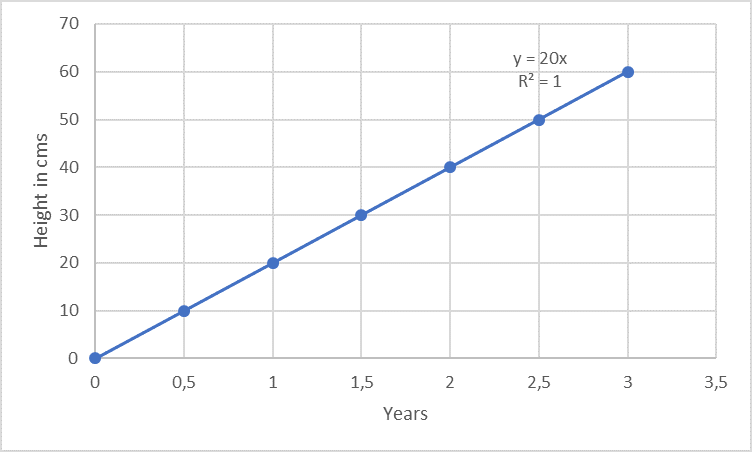# What is linearity?

Written by Anindya Ghosh Roy on . Posted in Method validation

In this small blog article, we would like to explain the parameter “Linearity” for an analytical method validation, its importance, and how it is calculated.

Mathematically speaking, linearity is a function of values that can be graphically represented as a straight line. Similarly, as per the method validation ICH Q2(R1) guideline, the linearity of an analytical method can be explained as its capability to show “results that are directly proportional to the concentration of the analyte in the sample”.

Linearity is often measured within a given range. For example, consider a plant that grows exactly 10 cm every 6 months for 3 years, the growth can be said to be linear for the 3 years.Unfortunately, the analytical response of a method is not always so distinctively linear as in the case of the growth of trees (example above).

Regarding analytical methods of drugs and active ingredients, the linearity of a signal can either be the relationship between the analyte’s signal and the analyte’s concentration in the calibration sample or in the sample matrix. The latter is more important because if the analyte’s signal within the sample is linear, it almost certain that it will also be the case in the calibration samples too. However, the same cannot be said for the opposite partly because of the matrix effect. A matrix effect is the influence caused by the other components of the sample to the expected response without the substance to be analyzed (e.g. formulation buffer). In case where the data are not linear, they might be mathematically transformed, e.g. applying logarithms but in some cases like in immunoassays no transformation won’t work at all. This is easy to understand for an ELISA if you consider the underlying principle of the method: a defined number of capture antibodies are bound in each well, which in turn can only bind a certain number of target proteins. This works well (i.e. there is a linear relationship) as long as free binding sites are still available. As soon as all binding sites are occupied, differences between different protein concentrations can’t be differentiated any longer due to the saturation effect!

Linearity is important for the confirmation of the method’s sensitivity for the analysis of the analyte’s concentration within a defined range. A defined range is important because for each drug the content of the active ingredient as well as the content of potential impurities is not always exactly identical from batch to batch, but can vary slightly, which is why concentrations above and below the respective expected value must also be determined correctly. As per the method validation ICH Q2(R1) guideline, linearity of a given response must be evaluated using at least a minimum of 5 concentrations of the analyte (multi-point calibration) and the data must be statistically analyzed, e.g. by performing regression analysis using the method of the least squares. The results are required to be represented in terms of the correlation coefficient R, Y-intercept, slope of the regression line and the residual sum of the squares in addition to graphical plotting. In a linear regression line y = mx + c, the regression coefficient is the constant “m” that represents the rate of change of one variable “y” as a function of change in the other “x” (thus the slope), while “c” is the Y-intercept. In simple terms, m is the slope and c is the ordinate intersection. But what do these two parameters like to tell us?

• If the regression line doesn’t pass through the origin (which is actually more or less almost always the case), i.e. we have a positive y-value at a concentration of 0, it provides us an indication of a constant systematic error (offset). In practical terms, this is the blank value, which is subtracted for correction.
• The slope, on the other hand, gives us information about the sensitivity of the method. It is easy to see that a steeper (i.e. greater) slope is associated with greater sensitivity, since it enables the discrimination of small differences in concentration. If, on the other hand, the slope is quite flat, small differences in concentration can’t be detected very well.

For a linear data set, calculation of the Pearson’s correlation coefficient R must prove to be sufficient. This coefficient is a value without unit telling us something about the degree of a linear relationship between two variables. If there is a perfect linear relationship, it has a value of 1. In case of a value being less than 0.95, it may either be a result of a broad spreading during measurement or due to a non-linear correlation. Often, the coefficient of determination (R2) is used, which is just the square of it. For most methods applied at least R2 ≥ 0.98 can be achieved.

It is mandatory for purity and assay methods (assays include content and potency determinations) to perform linearity studies for the validation of these methods. Linearity studies are important because they define the range of the method within which the results are obtained accurately and precisely. In case of impurities with very small amounts to be quantified, the needs to be evaluated. For the LOQ, trueness is also mandatory. Evaluating linearity near the LOQ (combined with repeatability and given specificity) can be used to infer trueness.

To summarize, linearity is one major aspect in the method validation procedure of assays and quantitative impurity tests. It provides to assess the range of concentrations for which the method can reliably function. For validation, multi-point calibration techniques are accepted, while single point calibrations are not. In case of a non-linear data set, higher order equations might be transformed, or the data must be accepted as they are while demonstrating a clear relation between analyte concentration and response.